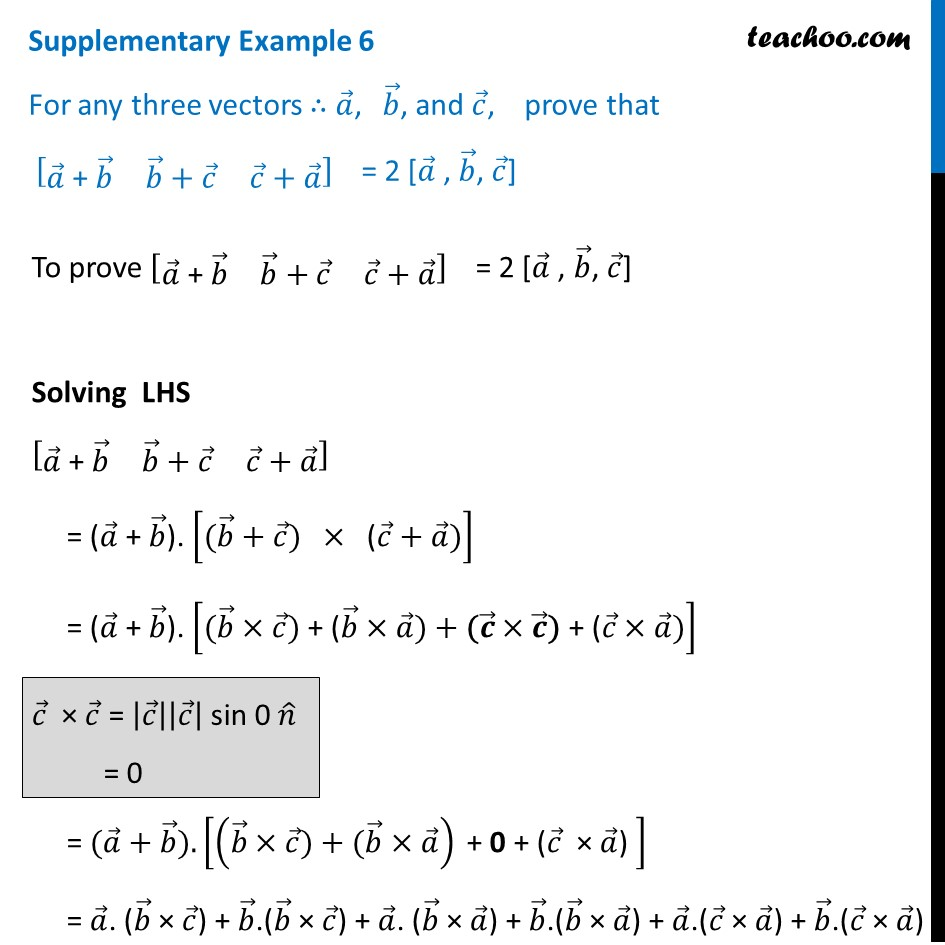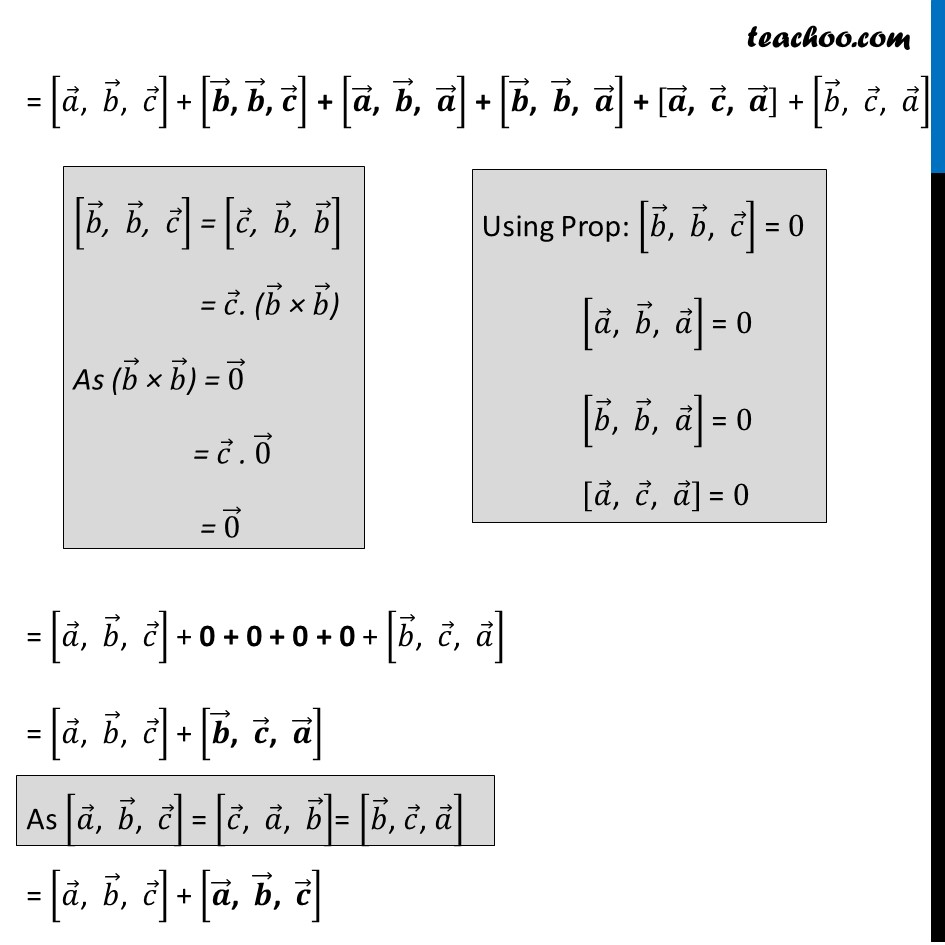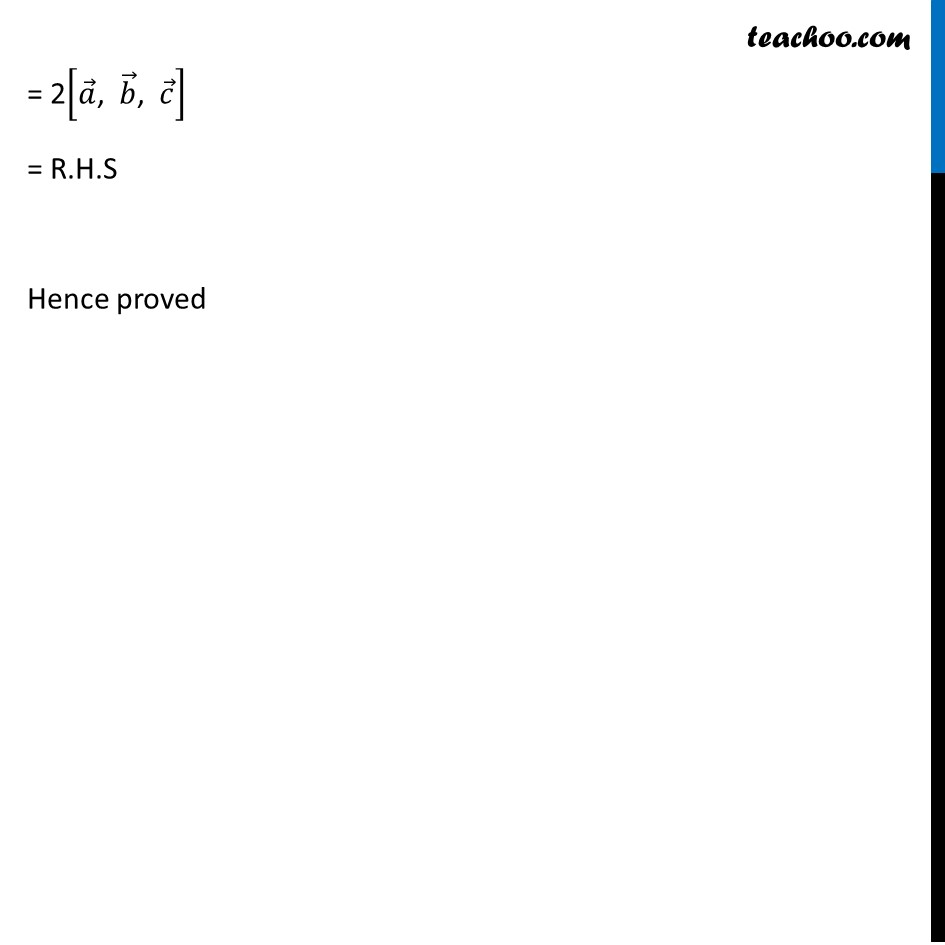Supplementary examples and questions from CBSE

Chapter 10 Class 12 Vector Algebra
Serial order wiseLearn in your speed, with individual attention - Teachoo Maths 1-on-1 Class

### Transcript

Supplementary Example 6 For any three vectors ∴ 𝑎 ⃗, 𝑏 ⃗, and 𝑐 ⃗, prove that [■8(𝑎 ⃗" + " 𝑏 ⃗&𝑏 ⃗+𝑐 ⃗&𝑐 ⃗+𝑎 ⃗ )] = 2 [𝑎 ⃗ , 𝑏 ⃗, 𝑐 ⃗] To prove [■8(𝑎 ⃗" + " 𝑏 ⃗&𝑏 ⃗+𝑐 ⃗&𝑐 ⃗+𝑎 ⃗ )] = 2 [𝑎 ⃗ , 𝑏 ⃗, 𝑐 ⃗] Solving LHS [■8(𝑎 ⃗" + " 𝑏 ⃗&𝑏 ⃗+𝑐 ⃗&𝑐 ⃗+𝑎 ⃗ )] = (𝑎 ⃗" + " 𝑏 ⃗). ["(" 𝑏 ⃗+𝑐 ⃗") " × " (" 𝑐 ⃗+𝑎 ⃗)] = (𝑎 ⃗" + " 𝑏 ⃗). ["(" 𝑏 ⃗×𝑐 ⃗") + (" 𝑏 ⃗×𝑎 ⃗)+"(" 𝒄 ⃗×𝒄 ⃗") + (" 𝑐 ⃗×𝑎 ⃗)] = "(" 𝑎 ⃗+𝑏 ⃗")." [(𝑏 ⃗×𝑐 ⃗") + (" 𝑏 ⃗×𝑎 ⃗ ) "+ 0 + (" 𝑐 ⃗" × " 𝑎 ⃗") " ] = 𝑎 ⃗. (𝑏 ⃗ × 𝑐 ⃗) + 𝑏 ⃗.(𝑏 ⃗ × 𝑐 ⃗) + 𝑎 ⃗. (𝑏 ⃗ × 𝑎 ⃗) + 𝑏 ⃗.(𝑏 ⃗ × 𝑎 ⃗) + 𝑎 ⃗.(𝑐 ⃗ × 𝑎 ⃗) + 𝑏 ⃗.(𝑐 ⃗ × 𝑎 ⃗) = [𝑎 ⃗", " 𝑏 ⃗", " 𝑐 ⃗ ] + [𝒃 ⃗", " 𝒃 ⃗", " 𝒄 ⃗ ] + [𝒂 ⃗", " 𝒃 ⃗", " 𝒂 ⃗ ] + [𝒃 ⃗", " 𝒃 ⃗", " 𝒂 ⃗ ] + [𝒂 ⃗", " 𝒄 ⃗", " 𝒂 ⃗ ] + [𝑏 ⃗", " 𝑐 ⃗", " 𝑎 ⃗ ] [𝑏 ⃗", " 𝑏 ⃗", " 𝑐 ⃗ ] = [𝑐 ⃗", " 𝑏 ⃗", " 𝑏 ⃗ ] = 𝑐 ⃗. (𝑏 ⃗ × 𝑏 ⃗) As (𝑏 ⃗ × 𝑏 ⃗) = 0 ⃗ = 𝑐 ⃗ . 0 ⃗ = 0 ⃗ Using Prop: [𝑏 ⃗", " 𝑏 ⃗", " 𝑐 ⃗ ] = 0 [𝑎 ⃗", " 𝑏 ⃗", " 𝑎 ⃗ ] = 0 [𝑏 ⃗", " 𝑏 ⃗", " 𝑎 ⃗ ] = 0 [𝑎 ⃗", " 𝑐 ⃗", " 𝑎 ⃗ ] = 0 = [𝑎 ⃗", " 𝑏 ⃗", " 𝑐 ⃗ ] + 0 + 0 + 0 + 0 + [𝑏 ⃗", " 𝑐 ⃗", " 𝑎 ⃗ ] = [𝑎 ⃗", " 𝑏 ⃗", " 𝑐 ⃗ ] + [𝒃 ⃗", " 𝒄 ⃗", " 𝒂 ⃗ ] = [𝑎 ⃗", " 𝑏 ⃗", " 𝑐 ⃗ ] + [𝒂 ⃗", " 𝒃 ⃗", " 𝒄 ⃗ ]